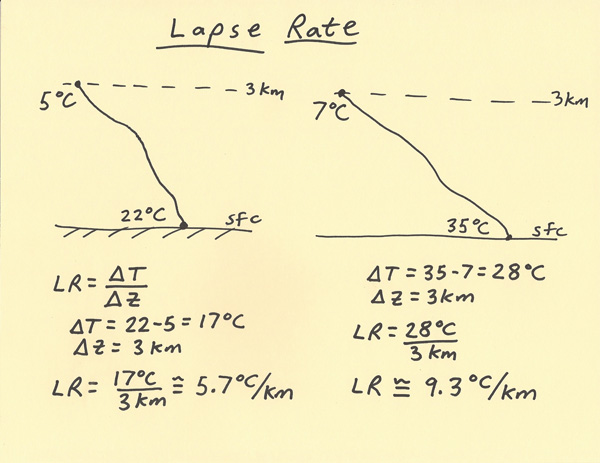[--MAIN HOME--] [--ALL HABYHINTS--] [--FACEBOOK PAGE--]

 LAPSE RATES

METEOROLOGIST JEFF HABY

The lapse rate is the change in temperature divided by the change in height. Lapse rates are important to weather forecasting since they help assess the (in)stability of layers in the troposphere. A higher number of lapse rate indicates a greater cooling with height. The typical situation is to have a lapse rate in which the temperature is decreasing with height but the temperature can increase with height such as with the case of an inversion. An inversion lapse rate can be indicated with a negative sign or a qualifier saying the temperature is increasing with height. A temperature increase with height is a very stable lapse rate in that layer.

The temperature difference, shown as delta T is found by finding the difference between the two temperature values at different elevations. On the diagram on left below, the temperature at the sfc is 22 C while the temperature at 3 km is 5 C. Thus, the temperature difference is 22 – 5 = 17. The change in height from the sfc to 700 mb is given as 3 km. The lapse rate is the temperature difference divided by the change in height which is 17 C / 3 C which results in a lapse rate of 5.7 C/km.

In the two examples below, temperature is decreasing with height. The diagram on the right (9.3 C/km) has a greater lapse rate as compared to the left diagram (5.7 C/km). As the lapse rate moves from 5 toward 10, the instability enhances. Weather forecasters look for high lapse rates to help forecast storms and severe weather.